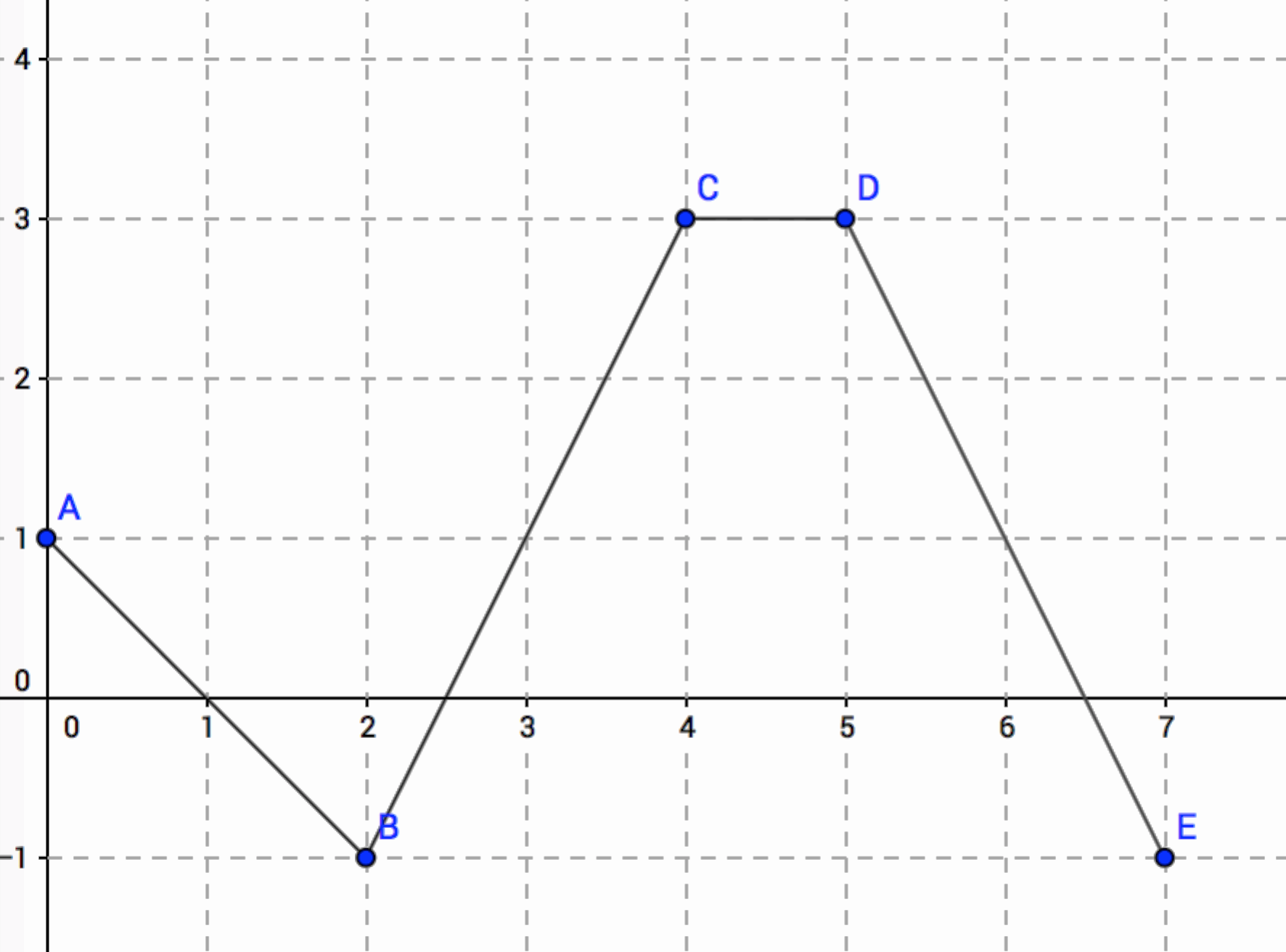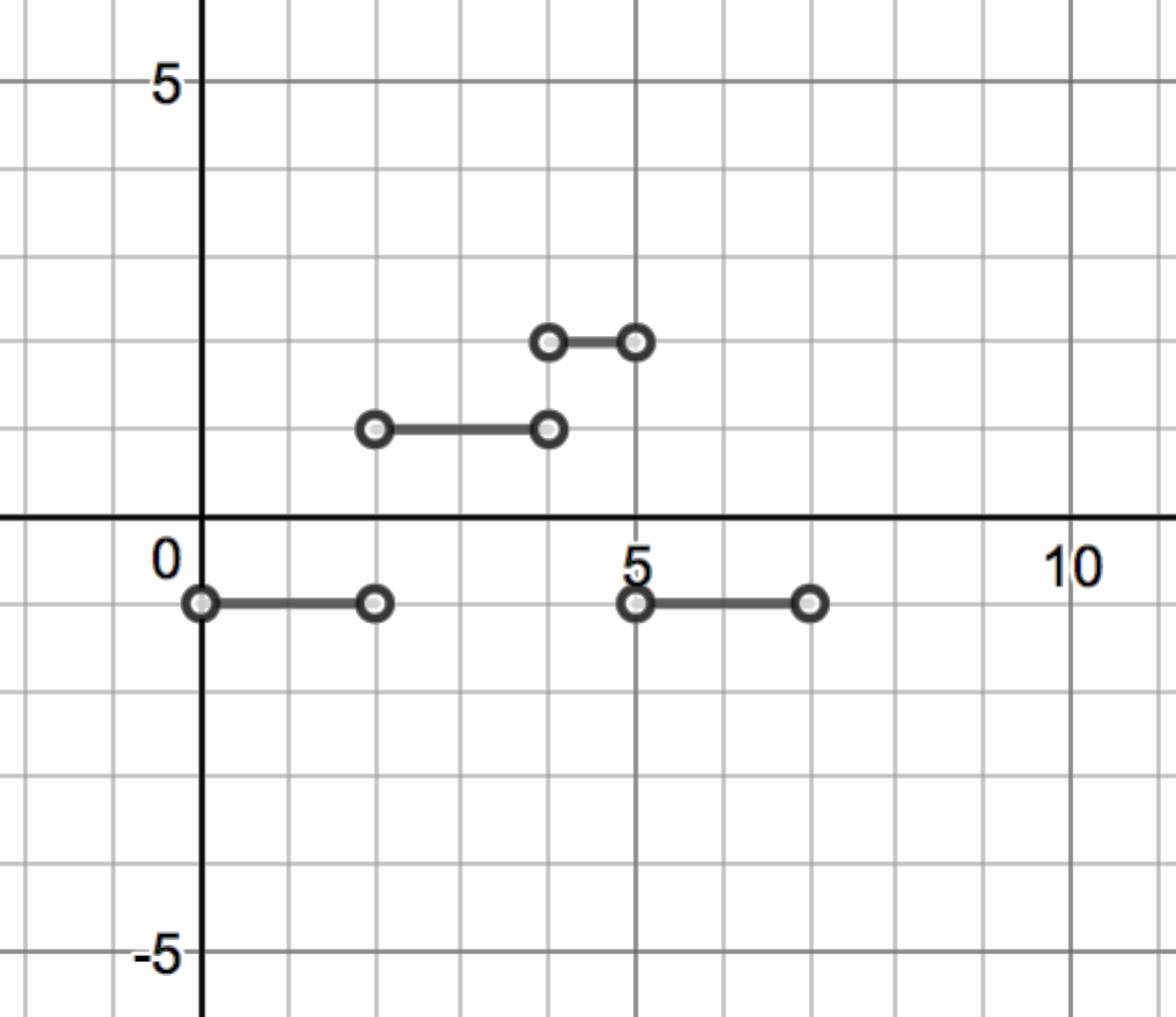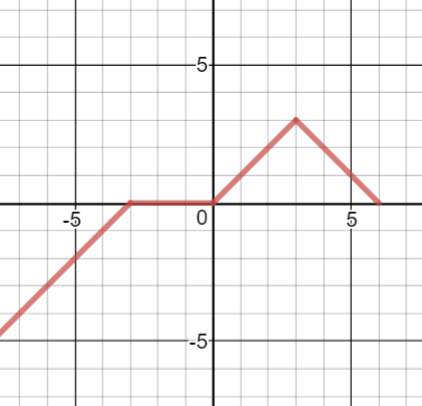# Limits and Continuity

## Objective

Sketch a slope graph from a linear piecewise function.

## Criteria for Success

?

1. Identify the slope for each piece of a continuous linear piecewise function.
2. Define the variables of a slope graph where $x$ is the input and $y$ is the rate of change not the value of the function.
3. Describe why the endpoints of the slope graph of a linear piecewise function are exclusive, that is, they are not included in the sub domain of the piece of the slope graph.

## Tips for Teachers

?

This lesson is aligned to the Learning Objectives and Essential Knowledge described in the College Board's AP Calculus AB and AP Calculus BC Course and Exam Description:

LO2.1A

LO2.1B (approaching)

## Anchor Problems

?

### Problem 1

Sketch a graph that shows the slope over each interval.#### References

Derivative of a linear piecewise function by cconrad is made available by GeoGebra under the CC BY-NC-SA 3.0 license. Copyright © International GeoGebra Institute, 2013. Accessed June 12, 2018, 11:52 a.m..

### Problem 2

Below is a sketch of a slope graph. Draw the sketch of the piecewise function.## Problem Set

?

The following resources include problems and activities aligned to the objective of the lesson that can be used to create your own problem set.

• Use this lesson to review the previous few lessons and incorporate a variety of practice.
• Give a piecewise function of the first derivative. Ask students to draw the graph of the first derivative and the original function.
• Find the slope graph from equations, without the graph.

Sketch the slope graph of ${{g(x)}}$. On which intervals is ${{g(x)}}$ increasing and decreasing?